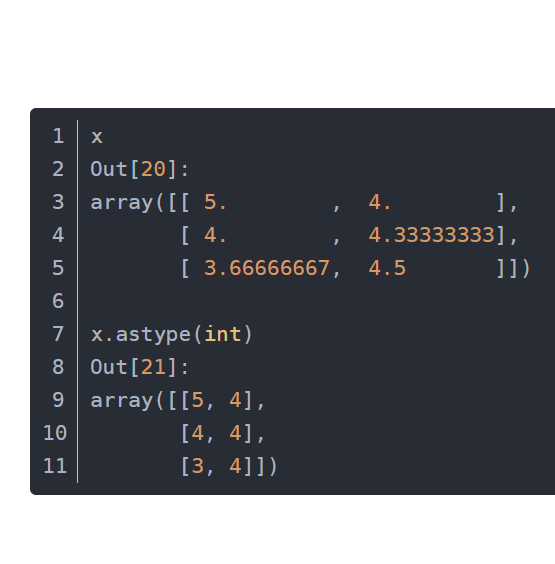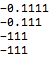• 怎么把小数换成整数
千次阅读
2019-01-04 16:30:26
Math.round(要转化的数);

这种方法是四舍五入的。

例如：var x1 = Math.round(11.3);

var x2 = Math.round(11.6);

那么结果为：x1 == 11

x2 == 12Math
更多相关内容
• 前言：当后台传输数据时传输的数字有可能是小数，但是客户要求必须显示在页面的是整数； 思路：因为后台传过来的数据大部分都是义以数组的形式传过来，将这些数据显示在页面要进行数据实例化并且页面要用for循环才...关于
• astype的返回值才是整数！！！ a = abs(np.random.normal(0, 2, size=30)) a = a.astype(int) !!!

astype的返回值才是整数！！！

a = abs(np.random.normal(0, 2, size=30))
a = a.astype(int)

!!!展开全文python
• 小数整数的话，要想进行转化，可以这个小数扩大10倍，再缩小10倍，然后进行计算。Q2：C语言如何提取出有限位小数小数部分并转化为整数？float f;scanf("%f", &f);printf("%d", (int)(f*100)%100);Q3：在...

快速导读：

Q1：小数乘整数如何转化的？

小数乘整数的话，要想进行转化，可以把这个小数扩大10倍，再缩小10倍，然后进行计算。

Q2：C语言如何提取出有限位小数的小数部分并转化为整数？

float f;

scanf("%f", &f);

printf("%d", (int)(f*100)%100);

Q3：在excel表格中如何将带小数的数值变成整数

假设数据在

A列

B1输入

=INT(A1)

鼠标移到B1

右下角

变实心

十字下拉

此公式

是去掉

小数位

，12.9也显示

12

如果是要四舍五入

就用

=ROUND(A1,0)

Q4：水晶报表公式字段怎么将小数化为整数

水晶报表取整的话貌似可以采用公式toText(round(value),0,'')就可以了，题主可以试试看哦。但是并不是很确定呢，毕竟很久没有用水晶报表了，现在常用的是finereport,直接进行设置小数点位数就可以了。

Q5：如何wwW.EcaibEI&.COm运用C#一个小数转化为整数

Math.Floor, Ceiling, Round方法总有一个合适的。

Q6：如何把小数系数化为整数系数 解方程(0？

全部乘以他们的最小公倍数，如100，那就是10x-20=2 希望我的回答能带给你帮助，给个好评吧亲！！

Q7：c++中如何将小数转化为整数

在所要求数字之前加 (int)

声明：该文观点仅代表作者本人，易采贝采用此文仅在于向读者提供更多信息，并不代表赞同其立场。

展开全文• #include &lt;iostream&gt; using namespace std; class Whole_to_decimal{ private: int x; public: Whole_to_decimal(int x_):x(x_){} float transfloat(){ int flag=0;... float t = ...
#include <iostream>
using namespace std;

class Whole_to_decimal{
private:
int x;
public:
Whole_to_decimal(int x_):x(x_){}
float transfloat(){
int flag=0;
float t = (float)x;
if(t<0)
{
t=-t;
flag=1;
}
while(t>1)
{
t/=10;
}
if(flag)
t=-t;
return t;
}
};
class Decimal_to_whole{
private:
float x;
public:
Decimal_to_whole(float x_):x(x_){}
int getWhole()
{
return (int)x;
}
float getdecimal()
{       float x_ = x;
int flag=0;
if(x_<0)
{
x_=-x_;
flag=1;
}
float decimal = (x_-(int)x_);
/*
当我用111.111-111后的结果是0.11100000697...末尾带有几个数
*/
if(flag)
decimal=-decimal;
return decimal;
}
int getdecimal2()
{
float x_=getdecimal();
int flag=0;
if(x_<0.0)
{
flag=1;
x_=-x_;
}
int t = 0;
while(x_>0.0)
{
x_*=10;
t+=(int)x_;
x_=(x_-(int)x_);

if(x_<=0.0001)
/*
如上，末尾带有几个无关的数，所以变不了0，只能取一个相对应的精度
*/
break;
t*=10;
}
if(flag)
t=-t;
return t;
}
};

int main()
{
Whole_to_decimal Test(-1111);
cout<<Test.transfloat()<<endl;
Decimal_to_whole Test2(-111.111);
cout<<Test2.getdecimal()<<endl;
cout<<Test2.getdecimal2()<<endl;
cout<<Test2.getWhole()<<endl;
}

结果：展开全文• mysql向数据库插入小数，变成了整数， 导致这个问题的原因是： 在设计表的时候，得定义表字段小数点的位数；应该设置小数位数 示例sql语句： CREATE TABLE MyClass ( id INT ( 4 ) NOT NULL PRIMARY KEY ...
• #include<stdio.h> int main() { int b=300; int m=47; for(int a=0;a<30;a++) { int c = b/m; printf("%d\n",c); int k =b%m*10; b=k;...一个计算器，符号优先级，正负数，小数转整
• ## java 中 小数转 整数

千次阅读 2020-05-28 16:24:25
Double d = 1.05; Integer i = d.intValue(); System.out.println(i); // 1
• a = [[1.,2.],[3.,4.]] b = torch.tensor(a) # c = torch.tensor(b,dtype=torch.int) c = b.clone().type(torch.int) print(b) print(c)pytorch python
• ## C# 小数转整数

千次阅读 2019-07-06 22:32:06
//遵循四舍五入原则： string test1="9.3"; string test2 = "9.5"; int int1= Convert.ToInt32(Convert.ToDouble(test1));...在C#中，要将一个字符串或浮点数转换为整数，基本上有三种方法： 1、使...
• ## SQL整数转换成小数

千次阅读 2021-01-21 16:21:25
2、然后可以通过round函数来进行小数处理，但是round会把小数后面变成0而不是真正意义上的保留位数。3、所有接下来就可以通过cast函数进行小数转化。4、但是在用cast的时候需要注意的是转化的类型中不要发生位数...
• //自动类型转型 ；赋值给对应的类型 #include <stdio.h> int main(){ // int代表着小数点去掉变成整数 int a=23.9; ...
• } //整数字符串格式检查 public static boolean StringIsInteger(String str){ return Pattern.matches("^(\\+|-)?[1-9]\\d*|0$", str); } //小数字符串格式检查 public static boolean StringIsDecimals(String ... • 在SQL Server中，将字符串的值转换为数值类型的值可以有三种方法。 1.使用cast()方法。...以上三种方法在转换类型的时候都要格外注意被转换类型的值，因为可能会出现各种强制转换失败的问题，比如小数sqlserver • ROUND(要舍入的字段,规定要返回的小数位数可以为0) • python 把带小数的浮点型字符串转换为整数的解决方案Python • 本节介绍浮点数和其他类型之间的相互转换，如将浮点数转换字符串或整数。由于 Python 是强类型语言，某些操作仅对特定类型有效，有时就需要先将...1) 转换整型——int()该函数将小数部分丢掉，该操作不会进行四... • 1.今天碰到一个需求，需要后台传来的某个有小数的字段转成整数。 2.解决方法 使用${#numbers.formatInteger(10.00,0)} 去掉小数部分即可。 ...thymeleaf
• 在项目中，计算出一个值为0.14，转化为字符串时...不甘心，测试了下从0.01到0.99转为整数的过程，果然不是单例。 好吧，我服了。for index in range(1,100,1): index = round(index / 100.0,2) print(index * 100)输python
• 1) 整数部分十进制整数转换为N进制整数采用“除N取余，逆序排列”法。具体做法是：将N作为除数，用十进制整数除以N，...先得到的余数作为N进制数的低位数字，后得到的余数作为N进制数的高位数字，依次排列起来，...
• 带小数点的字符串是不能直接转成整数的，应该先将它转成double类型再转int整数。 具体操作如下： String str = "80.0"; nt intValue = Double.valueOf(str).intValue();
• 在SQL Server中，将字符串的值转换为数值类型的值可以有三种方法。... -- 233 以上三种方法在转换类型的时候都要格外注意被转换类型的值，因为可能会出现各种强制转换失败的问题，比如小数位数/格式不匹配造成的报错。大数据
• ## 转: mysql的取整函数

千次阅读 2020-12-19 20:56:04
一、ROUND()函数用法ROUND(X) -- 表示将值 X 四舍五入为整数，无小数位ROUND(X,D) -- 表示将值 X 四舍五入为小数点后 D 位的数值，D为小数点后小数位数。若要保留 X 值小数点左边的 D 位，可将 D 设为负值。SELECT ...
• 问题描述： 直接使用int函数将字符串类型的小数转换为 int型会报错 问题解决： 先转换为float型，再转换为 int 型python
• sql server 整数转换成小数，并保留小数点后两位
• 在进行进制转换的时候，我们需要把小数分为整数小数两部分。 这里介绍两种方法。 第一种举个例子：1.23分为1 和 0.23 第二种：1.23 分为 1 和23 有时需要具体情况具体分析自己需要哪种类型。 第一种实现代码： ...
• 整数的字符串表示形式传递给int 将float的字符串表示形式传递给float 将整数的字符串表示形式传递给float 将一个浮球传递进去int 将整数传入float 但是，你得到一个ValueError，如果你传递的字符串表示浮到int...
• 1. 进制转换(整数间) ...间接法：十进制转二进制，然后再由二进制转八进制或者十六进制。 直接法：十进制转八进制或者十六进制按照除8或者16取余，直到商为0为止。 2. 进制转换(带小数点)带...
• 问题描述： 编写一个程序，当用户输入一个小数(正值浮点数)后，将小数转化为最近的整数输出（四舍五入）。
• 蒋俐祺【摘要】小数整数是在学生学习小数的意义和性质，小数的加法和减法的基础上进行教学的.四年级学习的积的变化规律也对本节课的教学有一定影响.学生在具体情境中探索并初步掌握小数整数的计算方法，会用竖式...c语言中小数乘整数...